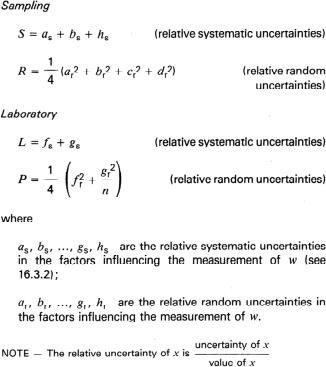ISO 3171 Petroleum liquids - Automatic pipeline sampling
16 Estimation of overall sampling system uncertainty
16.1 Introduction
This clause provides a mathematical procedure for estimating the overall uncertainty of the sampling system, taking into account the uncertainty of each element of the sampling and analytical sequence. The procedure uses an approximate formula (16.3) that can be applied in most cases.

The formula should be used by the design engineer to obtain information, at an early stage, concerning the overall uncertainty of the sampling system and the impact of each element on the final result. It thus provides an opportunity for technical and economic optimization.

The majority of the coefficients are determined by the construction characteristics of the system. These are not variable. The variables are the number of grabs taken N and the number of analyses n.

NOTES
1 A fundamental differente between error and uncertainty should be noted, which is that the former is by definition unknown, whereas the latter is an estimate.

2 Detailed information on how the formula is obtained will be given in ISO/TR 9494 (to be published).

16.2 Characteristics of sampling systems
The representativity of the sample obtained using an automatic sampler during a transfer depends on the arrangement and characteristics of the various elements used at different stages in the sampling sequence.

16.3 Formula for uncertainty calculations
The approximate formula referred to in 16.1 is as follows:where
w is the water content, as a percentage by volume;
D is the combined uncertainty associated with the random and systematic errors in w (95 % confidence level);
n is the number of analyses;
N is the number of grabs;
S, R, L and P are derived coefficients (see 16.3.1).

16.3.1 The derived coefficients are as follows:16.3.2 The factors influencing the measurement of w are as follows:
a) non-homogeneity of the water content as described clause 5 and annex A (for example poor dispersion):
relative systematic uncertainty as;
relative random uncertainty ar;

b) changes caused in the water content by the sampling system as described in clause 8 (for example non-isokinetic):
relative systematic uncertainty bs;
relative random uncertainty br;

c) uncertainty in the volume of each grab:
relative systematic uncertainty ds (not used, but see 16.4),
relative random uncertainty dr;

d) uncertainty in the flow rate caused by the flowmeter (degree of non-proportionality) as described in clause 10 (for example turbine meter factor variations):
relative systematic uncertainty cs (not used, but see 16.4),
relative random uncertainty cr;

e) changes in the water content during sampling as described in clause 11 (for example contamination or water vapour loss):
relative systematic uncertainty hs;
relative random uncertainty hr;

f) changes in the water content caused by sample handling and mixing as described in clause 12 (for example poor homogenization in the laboratory):
relative systematic uncertainty fs;
relative random uncertainty fr;

g) changes in the water content caused by transfer to Ia boratory glassware and analysis (for example centrifuge tube error):
relative systematic uncertainty gs;
relative random uncertainty gr.

16.4 Limitations of the formula
It is essential that the following conditions apply for the formula to be valid.
a) The number of grabs IV is greater than 1000.
b) The relative systematic uncertainty Cs, of the flowmeter in the working range is less than +/- 0.1 (i.e. less than 10 %).
c) The relative systematic uncertainty in the volume of the grab ds is less than +/- 0.1 (i.e. less than 10 %).

16.5 Examples
The examples which follow are designed to assist in applying the formula. The values used are typical ones taken from normal industrial practice.

1 7 Bibliography
ISO 3534, Statistics - Vocabulary and symbols.
ISO 3733, Petroleum products and bituminous materials - Determination of water - Distillation method.
ISO 3734, Crude petroleum and fuel oils - Determination of water and sediment - Centrifuge method.
ISO 3735, Crude petroleum and fuel oils - Determination of sediment - Extraction method.
ISO 5168, Measurement of fluid flow - Estimation of uncertainty of a flow-rate measurement.
ISO/TR 9494, Petroleum liquids - Automatic pipeline sampling - Statistical study of performance of automatic samplers